# 爬虫学习笔记（十七）—— 字符验证码

### 文章目录

• 一、认识验证码
• 1.1、概念
• 1.2、作用
• 1.3、类别
• 二、Pillow库
• 2.1、PIL库和Pillow库
• 2.1.1、Pillow库安装
• 2.1.2、PIL与Pillow使用注意
• 2.2、图形基本概念
• 2.2.1、尺寸
• 2.2.2、坐标系统
• 2.2.3、通道
• 2.3、图像获取
• 2.4、获取图像通道
• 三、简单验证码处理
• 3.1、灰度化
• 3.1.1、最大值法
• 3.1.2、平均值法
• 3.2、二值化
• 3.3、降噪
• 四、Tesseract识别
• 4.1、OCR识别概念
• 4.2、Tesseract-OCR
• 4.3、Pytesser3

# 二、Pillow库

## 2.1、PIL库和Pillow库

PIL库

PIL (Python Image Library) 已经算是 Python 处理图片的标准库了，兼具强大的功能和简洁的 API，但是PIL库的更新非常缓慢， 并且它只支持到python2.7，不支python3。

Pillow库

Pillow目前最新支持到python3.6，它的维护和开发十分活跃，兼容PIL库的绝大多数语法，并且增加了许多新的特性，推荐直接使用Pillow

### 2.1.1、Pillow库安装

``````pip install  pillow
``````

### 2.1.2、PIL与Pillow使用注意

Pillow和PIL不能共存在一个环境中，如果你之前安装了PIL的话，需要删除掉才能在安装Pillow

``````Import PIL
``````

## 2.2、图形基本概念

### 2.2.1、尺寸

``````from PIL import Image
img = Image.open('xxx.png')  #参数：图片位置
print(img.size)  #(xxx,xxx)
``````

### 2.2.2、坐标系统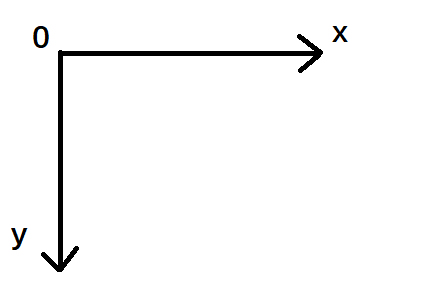### 2.2.3、通道

RGB图像：每张图片都是由三个数据通道叠加构成，分别为R 、G 、B。

PNG图像：有RGBA四个通道，A代表透明度。

## 2.3、图像获取

1. 从文件中加载图像

``````from PIL import Image
img = Image.open('dog2.png')  #open参数: 图片的位置
``````
1. 创建一个新的图像

``````img =Image.new("RGB",(200,100),"red")
``````

1. 切割图片

``````Image.crop(left, up, right, below)
``````

① left：与左边界的距离
② up：与上边界的距离
③ right：还是与左边界的距离
④ below：还是与上边界的距离

``````from PIL import Image

img = Image.open('dog2.png')
w,h = img.size

# 准备将图片切割成9张小图片
weight = int(w // 3)
height = int(h // 3)
# 切割后的小图的宽度和高度
print(weight, height)

for j in range(3):
for i in range(3):
box = (weight * i, height * j, weight * (i + 1), height * (j + 1))
region = img.crop(box)
region.save('imgs/{}{}.png'.format(j, i))
``````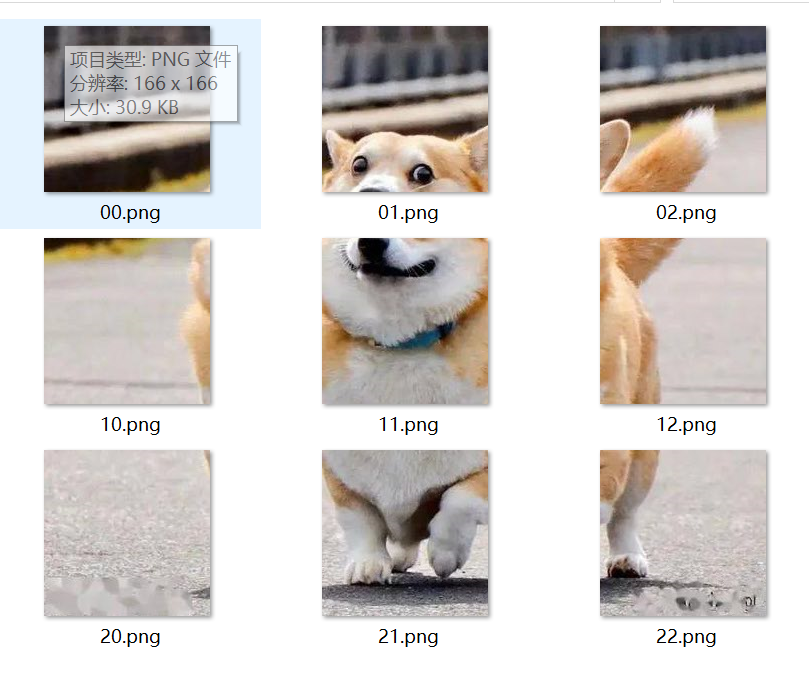## 2.4、获取图像通道

``````from PIL import Image

img = Image.open('dog2.png')
print(img.getbands())  # ('R', 'G', 'B')
``````

# 三、简单验证码处理

## 3.1、灰度化

`````` R=G=B = 处理前的 RX0.3 + GX0.59 + B*0.11
``````

### 3.1.2、平均值法

``````from PIL import Image

img = Image.open('code.jpeg')
img.show()
im = img.convert('L')  #转为灰度图
print(im.getbands())  #('L',)
im.show()
``````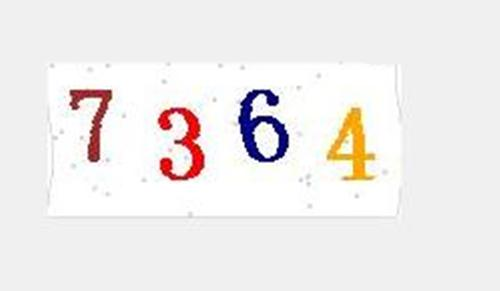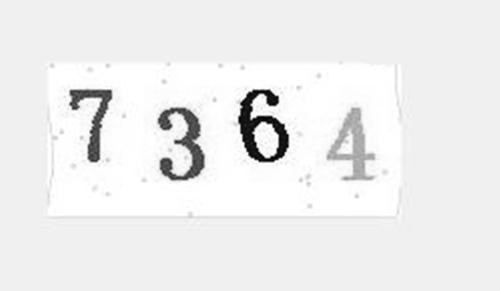## 3.2、二值化

• 灰度平均值值法： 取127 （0~255的中数， (0+255)/2 = 127）
• 平均值法：
计算像素点矩阵中的所有像素点的灰度值的平均值avg
• 迭代法：
选择一个近似阈值作为估计值的初始值（比如全图像的平均灰度），然后进行分割图像，产生两组像素，一组大于初始灰度值，另一组小于初始灰度值，根据产生的子图像的特征来选取新的阈值，在利用新的阈值分割图像，经过多次循环，使得错误分割的图像像素点降到最小。

``````def ImgBinaryzation(img_gray):  #灰度图二值化  传入的图片为灰度图
w,h=img_gray.size
temp = 0
for i in range(w):
for j in range(h):
temp += img_gray.getpixel((i,j))
# 阈值
avg_pixel = temp /(i*j)
#像素点和阈值比较
for x in range(w):
for y in range(h):
img_pixel = img_gray.getpixel((x,y))
if img_pixel < avg_pixel:  #小于像素点 像素点设为0
img_gray.putpixel((x,y),0)
else:   #不小于像素点 像素点设为255
img_gray.putpixel((x,y),255)
return img_gray
``````

``````from PIL import Image

img = Image.open('code.jpeg')
img_gray = img.convert('L')

def ImgBinaryzation(img_gray):  #灰度图二值化
w,h=img_gray.size
temp = 0
for i in range(w):
for j in range(h):
temp += img_gray.getpixel((i,j))
# 阈值  如果二值化效果不好，可以再根据结果乘以百分比
avg_pixel = temp /(i*j)
#像素点和阈值比较
for x in range(w):
for y in range(h):
img_pixel = img_gray.getpixel((x,y))
if img_pixel < avg_pixel:  #小于像素点 像素点设为0
img_gray.putpixel((x,y),0)
else:   #不小于像素点 像素点设为255
img_gray.putpixel((x,y),255)
return img_gray

pic = img_binaryzation(img_gray)
pic.show()
``````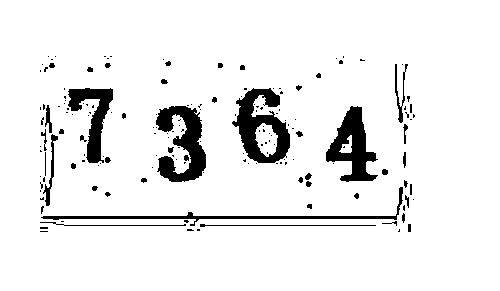## 3.3、降噪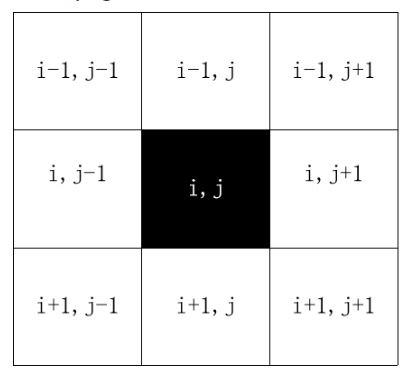``````def GetPointList(x,y,prange):  #获取像素点周围的坐标
for i in range(x-prange,x+prange+1):
for j in range(y-prange,y+prange+1):
if x==i and y == j:
continue
yield i,j

def reduceNoise(img_binary):  #二值化后再进行降噪
w,h = img_binary.size
prange = 3   #到边界的范围   自己根据降噪效果进行调试
bound_rate = 0.14  #设置边界比例   自己根据降噪效果进行调试
count_rate = 0.56  #设置像素点周围灰度值为255的比例    自己根据降噪效果进行调试
for i in range(w):
for j in range(h):
# 处理边界 变白
if i<bound_rate*w or i>w*(1 - bound_rate) or j<bound_rate*h or j>h*(1 - bound_rate):
img_binary.putpixel((i,j),255)
continue
img_pixel = img_binary.getpixel((i,j))
if img_pixel < 200:  #二值图像素点只有0和255，随便取个中间的值就行
count = 0
for x,y in GetPointList(i,j,prange):
if img_binary.getpixel((x,y)) >200:  #计算该像素点周围灰度值为255的个数
count += 1
if count > count_rate * ((prange*2+1)**2 - 2):  #像素点周围灰度值为255的个数是否超过一定比例来判断是否是噪点
img_binary.putpixel((i,j),255)
return img_binary
``````

``````from PIL import Image

img = Image.open('code.jpeg')
img_gray = img.convert('L')

def ImgBinaryzation(img_gray):  #灰度图二值化
w,h=img_gray.size
temp = 0
for i in range(w):
for j in range(h):
temp += img_gray.getpixel((i,j))
# 阈值
avg_pixel = temp /(i*j)
#像素点和阈值比较
for x in range(w):
for y in range(h):
img_pixel = img_gray.getpixel((x,y))
if img_pixel < avg_pixel:  #小于像素点 像素点设为0
img_gray.putpixel((x,y),0)
else:   #不小于像素点 像素点设为255
img_gray.putpixel((x,y),255)
return img_gray

def GetPointList(x,y,prange):  #获取像素点周围的坐标
for i in range(x-prange,x+prange+1):
for j in range(y-prange,y+prange+1):
if x==i and y == j:
continue
yield i,j

def reduceNoise(img_binary):  #二值化后再进行降噪
w,h = img_binary.size
prange = 3   #到边界的范围   自己根据降噪效果进行调试
bound_rate = 0.14  #设置边界比例   自己根据降噪效果进行调试
count_rate = 0.56  #设置像素点周围灰度值为255的比例    自己根据降噪效果进行调试
for i in range(w):
for j in range(h):
img_pixel = img_binary.getpixel((i,j))
if img_pixel < 200:  #二值图像素点只有0和255，随便取个中间的值就行
count = 0
for x,y in GetPointList(i,j,prange):
if img_binary.getpixel((x,y)) >200:  #计算该像素点周围灰度值为255的个数
count += 1
if count > count_rate * ((prange*2+1)**2 - 2):  #像素点周围灰度值为255的个数是否超过一定比例来判断是否是噪点
img_binary.putpixel((i,j),255)
return img_binary

pic = ImgBinaryzation(img_gray.copy())
for i in range(2):  #可能第一遍降噪效果不大会，进行多次处理
pic = reduceNoise(pic.copy())

pic.show()
``````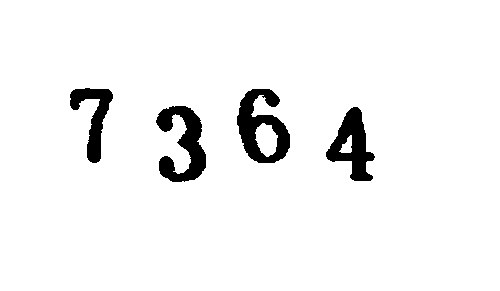# 四、Tesseract识别

## 4.1、OCR识别概念

OCR （Optical Character Recognition）光学字符识别， 指的是对文本资料的图像文件进行分析识别处理，获取文集及版面信息的过程

## 4.2、Tesseract-OCR

Windows下安装：

https://digi.bib.uni-mannheim.de/tesseract/ 可自行下载,点击下一步即可;

Linux安装：

``````sudo apt-get install tesseract-ocr
sudo apt-get install libtesseract-dev
``````

Mac安装：

``````brew install tesseract
``````

## 4.3、Pytesser3

Pytesseract文档：https://pypi.org/project/pytesseract/

``````pytesseract.pytesseract.tesseract_cmd = r'X:\xxx\tesseract.exe'
``````

``````import pytesseract
from PIL import Image

pic = Image.open('reduceNoise.png')  #图片为上面降噪结果图
print(pytesseract.image_to_string(pic))
# 结果：7364
``````

Pytesseract识别效果不佳，如果想提高识别率，可以使用jTessBoxEditor对Tesseract进行简单的训练。OCR是一个专门的图像处理的领域，高精度的识别需要依靠深度学习、神经网络等技术。

### 热门文章## Java输出数组的内容## 母螳螂的“魅惑之术”#win7 python3.7.0 import os,reos.chdir(d:\documents\program_language) file1open(.\疯狂填词_d9z9d2_r.txt) file2open(.\疯狂填词_d9z9d2_w.txt,w) words[ADJECTIVE,NOUN,VERB,NOUN] str1file1.read()#方法1 for word in words :word_replaceinput(fEnter a {word} :)str1…## 分享你的看书记录

1、软件评测师教程&#xff08;纸质版&#xff09;共19章 2022.01.16 第1-7章、15章看完## HBASE 高可用## js事件操作语法## Photoshop插件--晕影动态--选区--脚本开发--PS插件## SQL练习问题汇总_及时更新## vs LNK1104 无法打开文件“xxx.obj”## 工业元宇宙的定义与实施路线图## 【leectode 2022.1.15】完成一半题目## js 面试题总结## java练习代码## RocketMQ-什么是死信队列？怎么解决## 项目 cg day04## 输出三角形

#include <stdio.h> int main() { int i,j; for(i0;i<5;i) { for(j0;j<i;j) { printf("*"); } printf("\n"); } }## 通过awk和shell来限制IP多次访问之学不会你打死我## Python - 如何像程序员一样思考